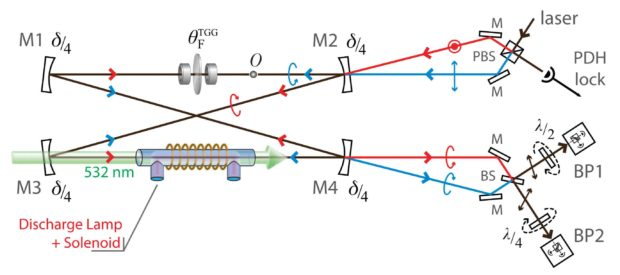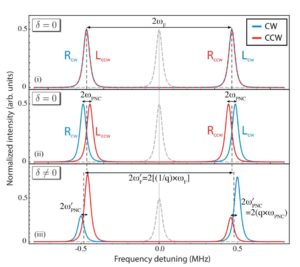# Fundamentals of cavity-enhanced polarimetry for parity-nonconserving optical rotation measurements: Application to Xe, Hg, and I

Physical Review A   89   052127 (2014)

L. Bougas, G. E. Katsoprinakis, W. von Klitzing, and T. Rakitzis

Abstract: We present the theoretical basis of a cavity-enhanced polarimetric scheme for the measurement of parity-nonconserving (PNC) optical rotation. We discuss the possibility of detecting PNC optical rotation in accessible transitions in metastable Xe and Hg, and ground state I. In particular, the physics of the PNC optical rotation is presented, and we explore the lineshape effects on the expected PNC optical rotation signals. Furthermore, we present an analysis of the eigenpolarizations of the cavity-enhanced polarimeter, which is necessary for understanding the measurement procedure and the ability of employing robust background subtraction procedures using two novel signal reversals. Using recent atomic structure theoretical calculations, we present simulations of the PNC optical rotation signals for all proposed transitions, assuming a range of experimentally feasible parameters. Finally, the possibility of performing sensitive measurements of the nuclear-spin-dependent PNC effects is investigated, for the odd-neutron nuclei 129Xe and 199Hg, and the odd-proton nucleus 127I.Cavity frequency polarization spectrum. For the simulations we used θF =13 mad and δ = θF/2 = 6.5 mad and for demonstration purposes φPNC = 0.6 mad. (i) The Faraday effect splits the cavity spectrum into R and L modes by 2ωF = 2θF(c/L) (twofold degeneracy); (ii) the PNC optical rotation splits further the clockwise (CW) and counterclockwise (CCW) modes by 2ωPNC = 2φPNC(c/L), while the cavity modes remain circular polarization states; (iii) in the presence of linear birefringence (δ ̸= 0) the frequency splitting of the eigenmodes increases as ωF′ = 1/qωF and the measured PNC- induced splitting is reduced ωF′ = qωF (0 ≤ q ≤ 1) (see Fig. 3); the eigenmodes transform into elliptical states as observed from the different amplitudes of the output light (see the text for discussion). We also assume that the clockwise input beam was p polarized, while the counterclockwise beam was s polarized. The cavity’s linewidth is exaggerated for demonstration purposes; note that the free spectral range of the cavity is ωFSR = 2π × 40 MHz. In (i)–(iii), the gray dashed line corresponds to the fourfold degenerate axial mode of an isotropic cavity. PBS stands for polarizing beam splitter and BS stands for beam splitter.